## Top-Level Model Coverage Report

If you simulate your model using the Run button, Simulink® Coverage™ creates a model coverage report for the specified model named `model_name_cov.html`. The model coverage report is also opened automatically in the Coverage Details pane. The model coverage report contains several sections:

To access the `sldemo_fuelsys` model, execute the following commands in the MATLAB® command window:

```addpath([matlabroot,'\examples\simulink_automotive\main']); open_system('sldemo_fuelsys');```

### Analysis Information

The analysis information section contains basic information about the model being analyzed:

• Coverage Data Information

• Model Information

• Harness Information (appears if you record coverage from a Simulink Test™ harness)

• Simulation Optimization Options

• Coverage Options### Aggregated Tests

The aggregated tests section appears if you:

• Record aggregated coverage results for at least two test cases through the Simulink Test Manager and produce a coverage report for the aggregated results, or

• Produce a coverage report for cumulative coverage results in the Results Explorer.

If you run test cases through the Simulink Test Manager, the aggregated tests section links to the associated test cases in the Simulink Test Manager.

If you aggregate test case results through the Results Explorer, the aggregated tests section links to the corresponding cvdata node in the Results Explorer.

For each run in the aggregated tests section, there is a link to the corresponding results in the Simulink Test Manager or the Results Explorer.

#### Aggregated Unit Tests

If you record coverage for one or more subsystem harnesses, the Aggregated Tests section lists each unit test run.

Each unit under test receives an ordinal number n, and each test for a unit under test receives an ordinal number m in the style `U`n.m.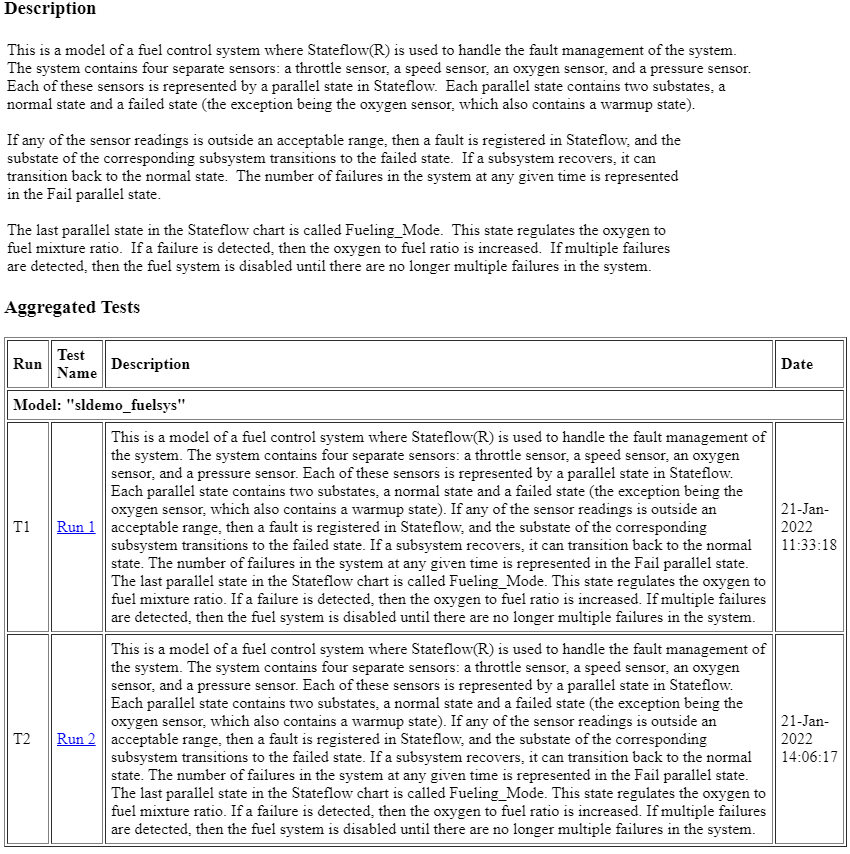### Coverage Summary

The coverage summary has two subsections:

• Tests — The simulation start and stop time of each test case and any setup commands that preceded the simulation. The heading for each test case includes any test case label specified using the `cvtest` command. This section only shows when the report does not contain an Aggregated Tests section.

• Summary — Summaries of the subsystem results. To see detailed results for a specific subsystem, in the Summary subsection, click the subsystem name.### Details

The Details section reports the detailed model coverage results. Each section of the detailed report summarizes the results for the metrics that test each object in the model:

You can also access a model element Details subsection as follows:

2. In the context menu, select Coverage > Report.

#### Filtered Objects

The Filtered Objects section lists all the objects in the model that were filtered from coverage recording, and the rationale you specified for filtering those objects. If the filter rule specifies that all blocks of a certain type be filtered, all those blocks are listed here.

In the following graphic, several blocks, subsystems, and transitions were filtered. Two library-linked blocks, protected division and protected division1, were filtered because their block library was filtered.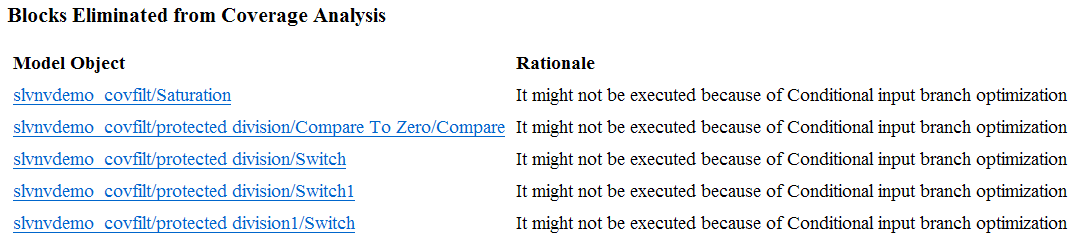#### Model Details

The Details section contains a results summary for the model as a whole, followed by a list of elements. Click the model element name to see its coverage results.

The following graphic shows the Details section for the `sldemo_fuelsys` example model.#### Subsystem Details

Each subsystem Details section contains a summary of the test coverage results for the subsystem and a list of the subsystems it contains. The overview is followed by sections for blocks, charts, and MATLAB functions, one for each object that contains a decision point in the subsystem.

The following graphic shows the coverage results for the Engine Gas Dynamics subsystem in the `sldemo_fuelsys` example model.#### Block Details

The following graphic shows decision coverage results for the MinMax block in the Mixing & Combustion subsystem of the Engine Gas Dynamics subsystem in the `sldemo_fuelsys` example model.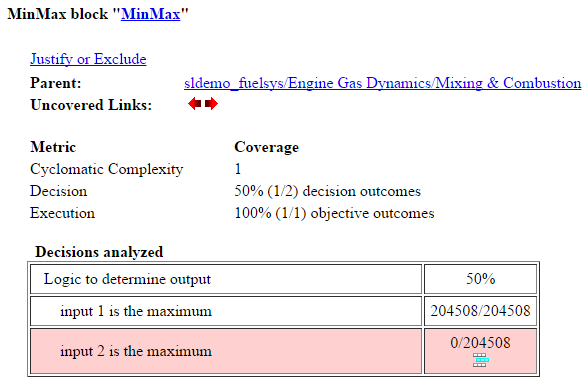The Uncovered Links element first appears in the Block Details section of the first block in the model hierarchy that does not achieve 100% coverage. The first Uncovered Links element has an arrow that links to the Block Details section in the report of the next block that does not achieve 100% coverage.

Subsequent blocks that do not achieve 100% coverage have links to the Block Details sections in the report of the previous and next blocks that do not achieve 100% coverage.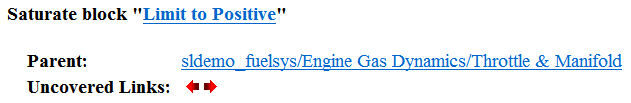#### Chart Details

The following graphic shows the coverage results for the Stateflow® chart `control_logic` in the `sldemo_fuelsys` example model.For more information about model coverage reports for Stateflow charts and their objects, see Model Coverage for Stateflow Charts.

#### Coverage Details for MATLAB Functions and Simulink Design Verifier Functions

By default, Simulink Coverage records coverage for all MATLAB functions in a model. MATLAB functions are in MATLAB Function blocks, Stateflow charts, or external MATLAB files.

Note

For a detailed example of coverage reports for external MATLAB files, see External MATLAB File Coverage Report.

To record Simulink Design Verifier™ coverage for `sldv.*` functions called by MATLAB functions, and any Simulink Design Verifier blocks, select Objectives and Constraints on the Coverage pane of the Configuration Parameters dialog box.

The following example shows coverage details for a MATLAB function, `hFcnsInExternalEML`, that calls four Simulink Design Verifier functions. In this example, the code for `hFcnsInExternalEML` resides in an external file.

This example also shows Simulink Design Verifier coverage details for the following functions:

In the coverage results, code that achieves 100% coverage is green. Code that achieves less than 100% coverage is red.Coverage for the `hFcnsInExternalEML` function and the `sldv.*` calls is:

• Line 1, the function declaration for `hFcnsInExternalEML`is green because the simulation executes that function at least once. `fcn` calls `hFcnsInExternalEML` 11 times during simulation.Line 4, `sldv.assume(u1 > u2)`, achieves 0% coverage because `u1 > u2` never evaluates to true.• Line 5, `sldv.condition(u1 == 0)`, achieves 100% coverage because `u1 == 0` evaluates to true for at least one time step.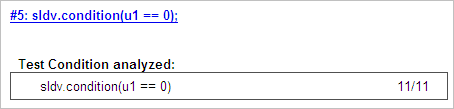• Line 6, `switch u1`, achieves 25% coverage because only one of the four outcomes in the `switch` statement (`case 0`) occurs during simulation.• Line 17, `sldv.test(y > u1); sldv.test (y == 4)` achieves 50% coverage. The first `sldv.test` call achieves 100% coverage, but the second `sldv.test` call achieves 0% coverage.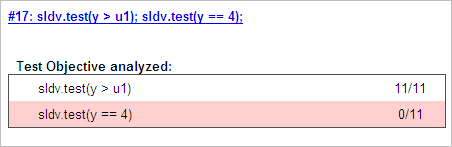#### Requirement Testing Details

If you run at least two test cases in Simulink Test that are linked to requirements in Simulink Requirements™, the aggregated coverage report details the links between model elements, test cases, and linked requirements.

The Requirement Testing Details section includes:

• Implemented Requirements — Which requirements are linked to the model element.

• Verified by Tests — Which tests verify the requirement.

• Associated Runs — Which runs are associated with each verification test.For an example of how to trace coverage results to requirements in a coverage report, see Trace Coverage Results to Requirements.

### Cyclomatic Complexity

You can specify that the model coverage report include cyclomatic complexity numbers in two locations in the report:

• The Summary section contains the cyclomatic complexity numbers for each object in the model hierarchy. For a subsystem or Stateflow chart, that number includes the cyclomatic complexity numbers for all their descendants.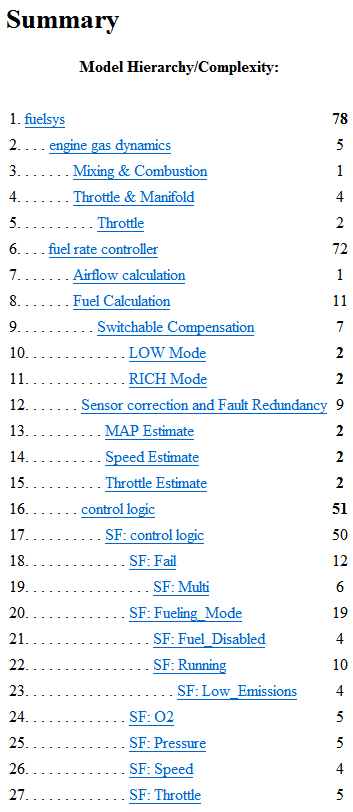• The Details sections for each object list the cyclomatic complexity numbers for all individual objects.### Decisions Analyzed

The Decisions analyzed table lists possible outcomes for a decision and the number of times that an outcome occurred in each test simulation. Outcomes that did not occur are in red highlighted table rows.

The following graphic shows the Decisions analyzed table for the Saturate block in the Throttle & Manifold subsystem of the Engine Gas Dynamics subsystem in the `sldemo_fuelsys` example model.To display and highlight the block in question, click the block name at the top of the section containing the block’s Decisions analyzed table.### Conditions Analyzed

The Conditions analyzed table lists the number of occurrences of true and false conditions on each input port of the corresponding block.### MCDC Analysis

The MCDC analysis table lists the MCDC input condition cases represented by the corresponding block and the extent to which the reported test cases cover the condition cases.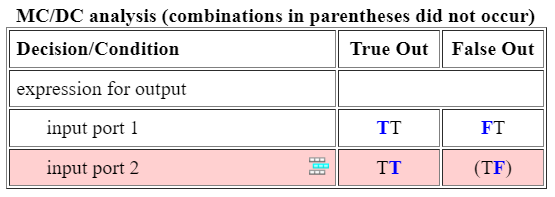Each row of the MCDC analysis table represents a condition case for a particular input to the block. A condition case for input `n` of a block is a combination of input values. Input `n` is called the deciding input of the condition case. Changing the value of input `n` alone changes the value of the block's output.

The MCDC analysis table shows a condition case expression to represent a condition case. A condition case expression is a character string where:

• The position of a character in the string corresponds to the input port number.

• The character at the position represents the value of the input. (`T` means `true`; `F` means `false`).

• A boldface character corresponds to the value of the deciding input.

For example, `F``T``F` represents a condition case for a three-input block where the second input is the deciding input.

The Decision/Condition column specifies the deciding input for an input condition case. The True Out column specifies the deciding input value that causes the block to output a `true` value for a condition case. The True Out entry uses a condition case expression, for example, `F``F`, to express the values of all the inputs to the block, with the value of the deciding variable in bold.

Parentheses around the expression indicate that the specified combination of inputs did not occur during the first (or only) test case included in this report. In other words, the test case did not cover the corresponding condition case. The False Out column specifies the deciding input value that causes the block to output a false value and whether the value actually occurred during the first (or only) test case included in the report.

Some model elements achieve less MCDC coverage depending on the MCDC definition used during analysis. For more information on how the MCDC definition used during analysis affects the coverage results, see Modified Condition and Decision Coverage (MCDC) Definitions in Simulink Coverage.

If you select Treat Simulink Logic blocks as short-circuited in the Coverage pane in the Configuration Parameters dialog box, MCDC coverage analysis does not verify whether short-circuited inputs actually occur. The MCDC analysis table uses an `x` in a condition expression (for example, `TFxxx`) to indicate short-circuited inputs that were not analyzed by the tool.

If you disable this feature and Logic blocks are not short-circuited while collecting model coverage, you might not be able to achieve 100% coverage for that block.

Select the Treat Simulink Logic blocks as short-circuited option for where you want the MCDC coverage analysis to approximate the degree of coverage that your test cases achieve for the generated code (most high-level languages short-circuit logic expressions).

### Cumulative Coverage

After you record successive coverage results, you can Access, Manage, and Aggregate Coverage Results from within the Coverage Results Explorer. By default, the results of each simulation are saved and recorded cumulatively in the report.

If you select Show cumulative progress report in the Results section of the configuration parameters, the results located in the right-most area in all tables of the cumulative coverage report reflect the running total value. The report is organized so that you can easily compare the additional coverage from the most recent run with the coverage from all prior runs in the session.

A cumulative coverage report contains information about:

• Current Run — The coverage results of the simulation just completed.

• Delta — Percentage of coverage added to the cumulative coverage achieved with the simulation just completed. If the previous simulation's cumulative coverage and the current coverage are nonzero, the delta may be 0 if the new coverage does not add to the cumulative coverage.

• Cumulative — The total coverage collected for the model up to, and including, the simulation just completed.

After running three test cases, the Summary report shows how much additional coverage the third test case achieved and the cumulative coverage achieved for the first two test cases.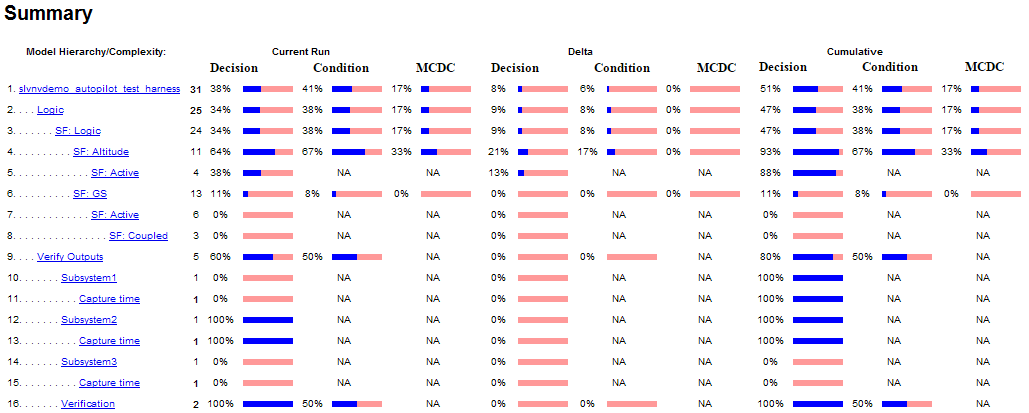The Decisions analyzed table for cumulative coverage contains three columns of data about decision outcomes that represent the current run, the delta since the last run, and the cumulative data, respectively.The Conditions analyzed table uses column headers #n T and #n F to indicate results for individual test cases. The table uses Tot T and Tot F for the cumulative results. You can identify the true and false conditions on each input port of the corresponding block for each test case.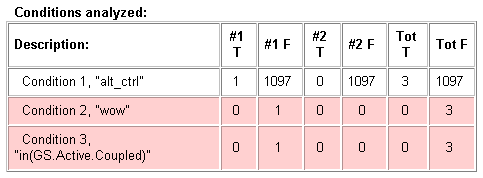The MCDC analysis #n True Out and #n False Out columns show the condition cases for each test case. The Total Out T and Total Out F column show the cumulative results.Note

You can calculate cumulative coverage for reusable subsystems and Stateflow constructs at the command line. For more information, see Obtain Cumulative Coverage for Reusable Subsystems and Stateflow® Constructs.

### N-Dimensional Lookup Table

The following interactive chart summarizes the extent to which elements of a lookup table are accessed. In this example, two Sine Wave blocks generate x and y indices that access a 2-D Lookup Table block of 10-by-10 elements filled with random values.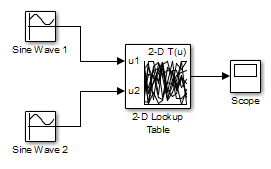In this model, the lookup table indices are 1, 2,..., 10 in each direction. The Sine Wave 2 block is out of phase with the Sine Wave 1 block by pi/2 radians. This generates x and y numbers for the edge of a circle, which you see when you examine the resulting Lookup Table coverage.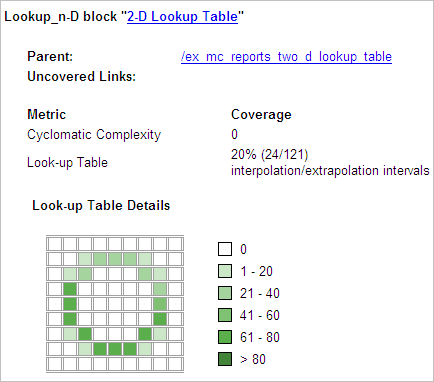The report contains a two-dimensional table representing the elements of the lookup table. The element indices are represented by the cell border grid lines, which number 10 in each dimension. Areas where the lookup table interpolates between table values are represented by the cell areas. Areas of extrapolation left of element 1 and right of element 10 are represented by cells at the edge of the table, which have no outside border.

The number of values interpolated (or extrapolated) for each cell (execution counts) during testing is represented by a shade of green assigned to the cell. Each of six levels of green shading and the range of execution counts represented are displayed on one side of the table.

If you click an individual table cell, you see a dialog box that displays the index location of the cell and the exact number of execution counts generated for it during testing. The following example shows the contents of a color-shaded cell on the right edge of the circle.The selected cell is outlined in red. You can also click the extrapolation cells on the edge of the table.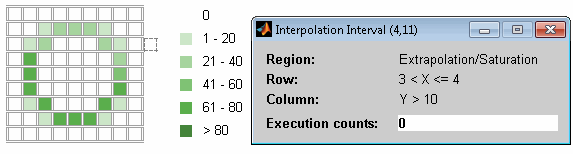A bold grid line indicates that at least one block input equal to its exact index value occurred during the simulation. Click the border to display the exact number of hits for that index value.The following example model uses an n-D Lookup Table block of 10-by-10-by-5 elements filled with random values.Both the x and y table axes have the indices 1, 2,..., 10. The z axis has the indices 10, 20,..., 50. Lookup table values are accessed with x and y indices that the two Sine Wave blocks generated, in the preceding example, and a z index that a Ramp block generates.

After simulation, you see the following lookup table report.Instead of a two-dimensional table, the link `Force Map Generation` displays the following tables: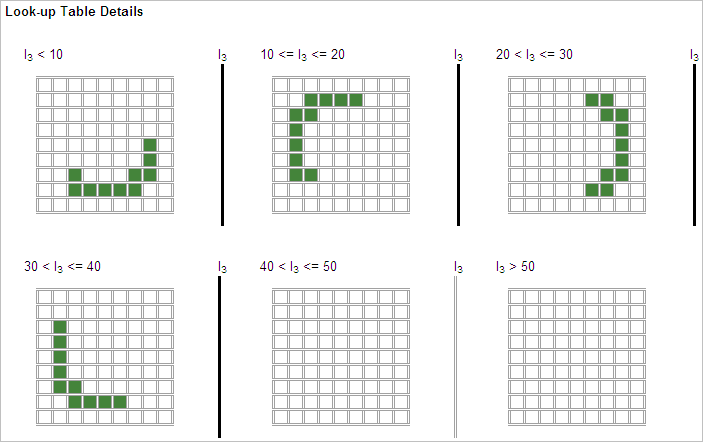Lookup table coverage for a three-dimensional lookup table block is reported as a set of two-dimensional tables.

The vertical bars represent the exact z index values: 10, 20, 30, 40, 50. If a vertical bar is bold, this indicates that at least one block input was equal to the exact index value it represents during the simulation. Click a bar to get a coverage report for the exact index value that bar represents.

You can report lookup table coverage for lookup tables of any dimension. Coverage for four-dimensional tables is reported as sets of three-dimensional sets, like those in the preceding example. Five-dimensional tables are reported as sets of sets of three-dimensional sets, and so on.

### Block Reduction

All model coverage reports indicate the status of the Simulink Block reduction parameter at the beginning of the report. In the following example, you set Force block reduction off.In the next example, you enabled the Simulink Block reduction parameter, and you did not set Force block reduction off.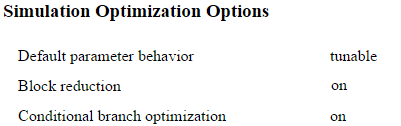Consider the following model where the simulation does not execute the MinMax1 block because there is only one input — `In3`.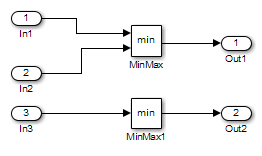If you set Force block reduction off, the report contains no coverage data for this block because the minimum input to the MinMax1 block is always `1`.

If you do not set Force block reduction off, the report contains no coverage data for reduced blocks.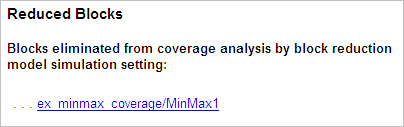### Relational Boundary

On the Coverage Pane of the Configuration Parameters dialog box, if you select the Relational Boundary coverage metric, the software creates a Relational Boundary table in the model coverage report for each model object that is supported for this coverage. The table applies to the explicit or implicit relational operation involved in the model object. For more information, see:

The tables below show the relational boundary coverage report for the relation `input1 <= input2`. The appearance of the tables depend on the operand data type.

#### Integers

If both operands are integers (or if one operand is an integer and the other a Boolean), the table appears as follows.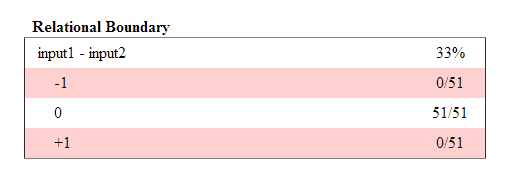For a relational operation such as ```operand_1 <= operand_2```:

• The first row states the two operands in the form ```operand_1 - operand_2```.

• The second row states the number of times during the simulation that ```operand_1 - operand_2``` is equal to -1.

• The third row states the number of times during the simulation that `operand_1` is equal to `operand_2`.

• The fourth row states the number of times during the simulation that ```operand_1 - operand_2``` is equal to 1.

#### Fixed point

If one of the operands has fixed-point type and the other operand is either a fixed point or an integer, the table appears as follows. `LSB` represents the value of the least significant bit. For more information, see Precision (Fixed-Point Designer). If the two operands have different precision, the smaller value of precision is used.For a relational operation such as ```operand_1 <= operand_2```:

• The first row states the two operands in the form ```operand_1 - operand_2```.

• The second row states the number of times during the simulation that ```operand_1 - operand_2``` is equal to `-LSB`.

• The third row states the number of times during the simulation that `operand_1` is equal to `operand_2`.

• The fourth row states the number of times during the simulation that ```operand_1 - operand_2``` is equal to `LSB`.

#### Floating point

If one of the operands has floating-point type, the table appears as follows. `tol` represents a value computed using the input values and a tolerance that you specify. If you do not specify a tolerance, the default values are used. For more information, see Relational Boundary Coverage.For a relational operation such as ```operand_1 <= operand_2```:

• The first row states the two operands in the form ```operand_1 - operand_2```.

• The second row states the number of times during the simulation that ```operand_1 - operand_2``` has values in the range `[-tol..0]`.

• The third row states the number of times during the simulation that ```operand_1 - operand_2``` has values in the range `(0..tol]` during the simulation.

The appearance of this table changes according to the relational operator in the block. Depending on the relational operator, the value of ```operand_1 - operand_2``` equal to 0 is either:

• Excluded from relational boundary coverage.

• Included in the region above the relational boundary.

• Included in the region below the relational boundary.

Relational OperatorReport FormatExplanation
`==``[-tol..0)`0 is excluded.
`(0..tol]`
`!=``[-tol..0)`0 is excluded.
`(0..tol]`
`<=``[-tol..0]`0 is included in the region below the relational boundary.
`(0..tol]`
`<``[-tol..0)`0 is included in the region above the relational boundary.
`[0..tol]`
`>=``[-tol..0)`0 is included in the region above the relational boundary.
`[0..tol]`
`>``[-tol..0]`0 is included in the region below the relational boundary.
`(0..tol]`

0 is included below the relational boundary for `<=` but above the relational boundary for `<`. This rule is consistent with decision coverage. For instance:

• For the relation `input1 <= input2`, the decision is true if `input1` is less than or equal to `input2`. `<` and `=` are grouped together. Therefore, 0 lies in the region below the relational boundary.

• For the relation `input1 < input2`, the decision is true only if `input1` is less than `input2`. `>` and `=` are grouped together. Therefore, 0 lies in the region above the relational boundary.

### Saturate on Integer Overflow Analysis

On the Coverage Pane of the Configuration Parameters dialog box, if you select the Saturate on integer overflow coverage metric, the software creates a Saturation on Overflow analyzed table in the model coverage report. The software creates the table for each block with the Saturate on integer overflow parameter selected.

The Saturation on Overflow analyzed table lists the number of times a block saturates on integer overflow, indicating a true decision. If the block does not saturate on integer overflow, the table indicates a false decision. Outcomes that do not occur are in red highlighted table rows.

The following graphic shows the Saturation on Overflow analyzed table for the MinMax block in the Mixing & Combustion subsystem of the Engine Gas Dynamics subsystem in the `sldemo_fuelsys` example model.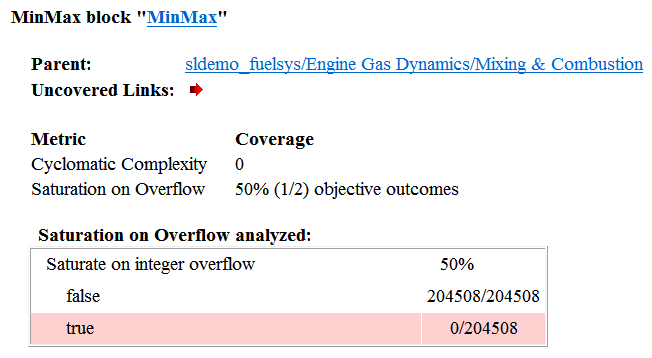To display and highlight the block in question, click the block name at the top of the section containing the block’s Saturation on Overflow analyzed table.### Signal Range Analysis

If you select the Signal Range coverage metric, the software creates a Signal Range Analysis section at the bottom of the model coverage report. This section lists the maximum and minimum signal values for each output signal in the model measured during simulation.

Access the Signal Range Analysis report quickly with the Signal Ranges link in the nonscrolling region at the top of the model coverage report, as shown below in the `sldemo_fuelsys` example model report.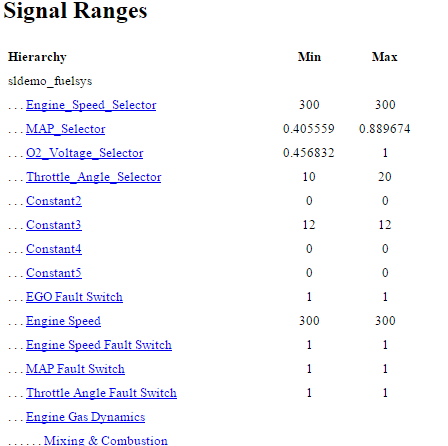Each block is reported in hierarchical fashion; child blocks appear directly under parent blocks. Each block name in the Signal Ranges report is a link. For example, select the `EGO sensor` link to display this block highlighted in its native diagram.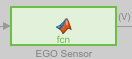### Signal Size Coverage for Variable-Dimension Signals

If you select Signal Size, the software creates a Variable Signal Widths section after the Signal Ranges data in the model coverage report. This section lists the maximum and minimum signal sizes for all output ports in the model that have variable-size signals. It also lists the memory that Simulink allocated for that signal, as measured during simulation. This list does not include signals whose size does not vary during simulation.

The following example shows the Variable Signal Widths section in a coverage report. In this example, the Abs block signal size varied from 2 to 5, with an allocation of 5.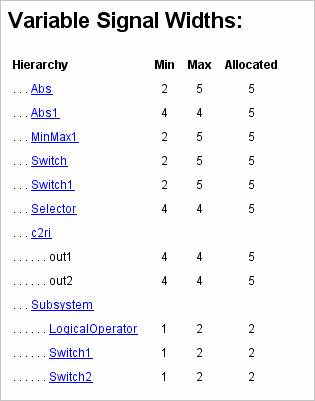Each block is reported in hierarchical fashion; child blocks appear directly under parent blocks. Each block name in the Variable Signal Widths list is a link. Clicking on the link highlights the corresponding block in the Simulink Editor. After the analysis, the variable-size signals have a wider line design.

If you select Objectives and Constraints, the analysis collects coverage data for all Simulink Design Verifier blocks in your model.

For an example of how this works, open the `sldvdemo_debounce_testobjblks` model.

This model contains two Test Objective blocks:

• The True block defines a property that the signal have a value of `2`.

• The Edge block, inside the Masked Objective subsystem, describes the property where the output of the AND block in the Masked Objective subsystem changes from `2` to `1`.

The Simulink Design Verifier software analyzes this model and produces a harness model that contains test cases that achieve certain test objectives. To see if the original model achieves those objectives, simulate the harness model and collect model coverage data. The model coverage tool analyzes any decision points or values within an interval that you specify in the Test Objective block.

In this example, the coverage report shows that you achieved 100% coverage of the True block because the signal value was `2` at least once. The signal value was `2` in 6 out of 14 time steps.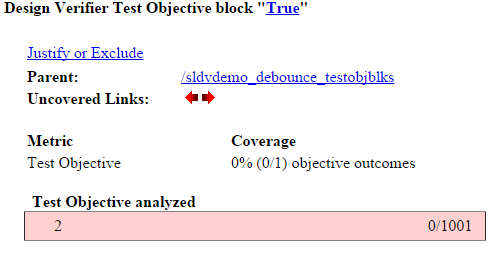The input signal to the Edge block achieved a value of `True` once out of 14 time steps.